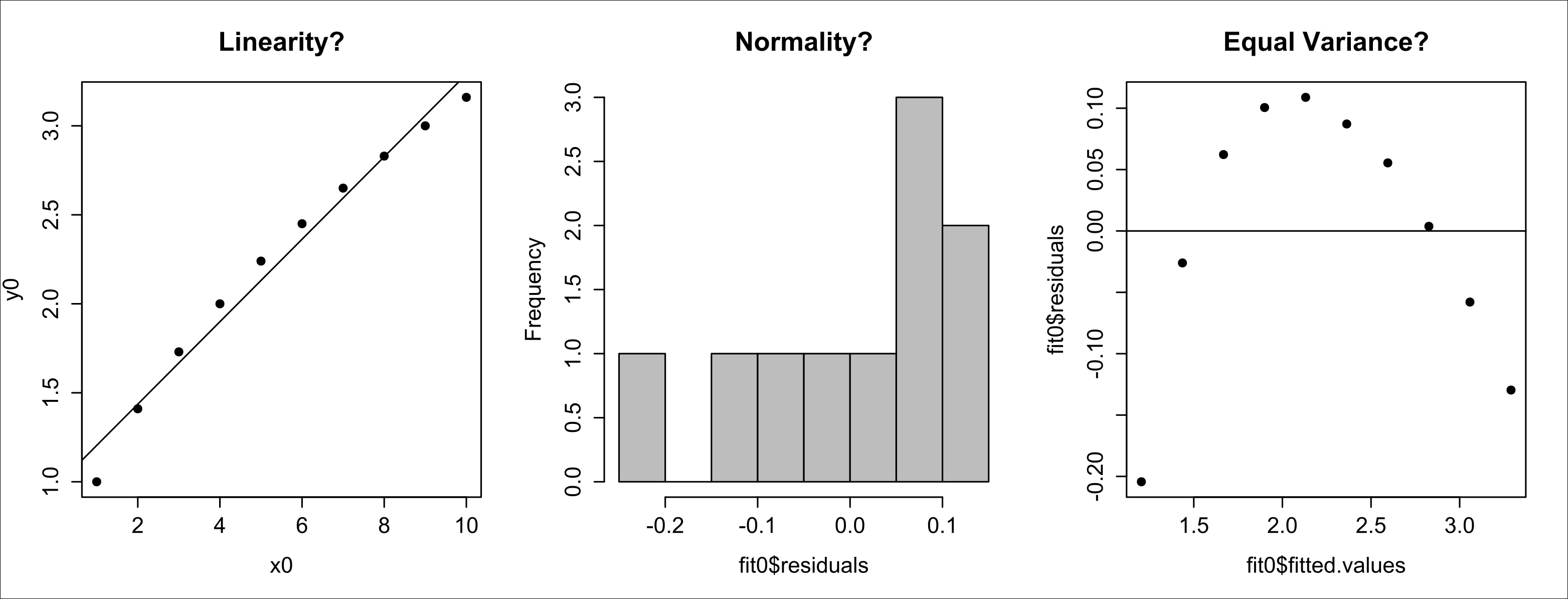•#### Introduction to R for Business Intelligence#### Overview of this book

Introduction to R for Business IntelligenceCreditsAcknowledgementwww.PacktPub.comPrefaceFree ChapterData CleaningExploratory Data AnalysisData Mining with Cluster AnalysisTime Series AnalysisVisualizing the Datas StoryWeb Dashboards with ShinyReferencesR Packages Used in the BookR Code for Supporting Market Segment Business Case Calculations## Refining data for simple linear regression

As discussed earlier, there may be times when your diagnostic plots indicate that the data does not meet all the assumptions specified by the LINE approach (Linearity, Independence, Normality, and Equal variance).

Consider the following simple dataset. Run a SLR and generate its diagnostic plots:

```x0 <- c(1, 2, 3, 4, 5, 6, 7, 8, 9, 10)
y0 <- c(1.00, 1.41, 1.73, 2.00, 2.24,
2.45, 2.65, 2.83, 3.00, 3.16)
fit0 <- lm(y0 ~ x0)

par(mfrow = c(1, 3))
plot(x0, y0, pch = 19, main = "Linearity?"); abline(fit0)
hist(fit0\$residuals, main = "Normality?", col = "gray")
plot(fit0\$fitted.values, fit0\$residuals,
main = "Equal Variance?", pch = 19); abline(h = 0)
```

The diagnostic plots generated are as follows:The first plot shows some deviation from linearity. Recall the saying If things look OK, then they probably are OK. You are going to focus on the other two plots, which do not meet the assumptions of normality or equal variance...